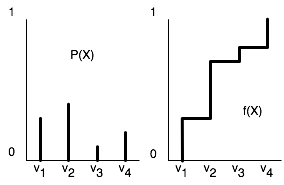#### 6.4.2.1 Sampling from a Single Variable

To generate samples from a single discrete or real-valued variable, X, first totally order the values in the domain of X. For discrete variables, if there is no natural order, you can just create an arbitrary ordering. Given this ordering, the cumulative probability distribution is a function of x, defined by f(x)=P(X ≤ x).Figure 6.9: A cumulative probability distribution

To generate a random sample for X, select a random number y in the range [0,1]. We select y from a uniform distribution to ensure that each number between 0 and 1 has the same chance of being chosen. Let v be the value of X that maps to y in the cumulative probability distribution. That is, v is the element of dom(X) such that f(v)=y or, equivalently, v=f-1(y). Then, X=v is a random sample of X, chosen according to the distribution of X.

Example 6.22: Consider a random variable X with domain {v1,v2,v3,v4}. Suppose P(X=v1)= 0.3, P(X=v2)=0.4, P(X=v3)=0.1, and P(X=v4)=0.2. First, totally order the values, say v1 < v2 < v3 < v4. Figure 6.9 shows P(X), the distribution for X, and f(X), the cumulative distribution for X. Consider value v1; 0.3 of the range of f maps back to v1. Thus, if a sample is uniformly selected from the Y-axis, v1 has a 0.3 chance of being selected, v2 has a 0.4 chance of being selected, and so forth.